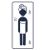# Rentals close to the slopes - Page 14

Viewing mode
350 results
• Slopes access :
• ski-in (-50 m)
• Rooms :
• Studio
• Total surface (sq.m) :
• 30 sq.m
•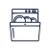•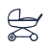•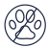• Slopes access :
• ski-in (-50 m)
• Rooms :
• Studio
• Studio + cabin(s)
• Total surface (sq.m) :
• 35 sq.m
•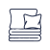•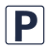•••• Slopes access :
• ski-in (-50 m)
• Rooms :
• 2 rooms
• Total surface (sq.m) :
• 35 sq.m
•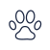••• Slopes access :
• ski-in (-50 m)
• Total surface (sq.m) :
• 29 sq.m
•••••• Slopes access :
• ski-in (-50 m)
• Rooms :
• Studio + cabin(s)
• Total surface (sq.m) :
• 25 sq.m
••• Slopes access :
• ski-in (-50 m)
• Rooms :
• 2 rooms
• Total surface (sq.m) :
• 52 sq.m
••••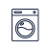• Slopes access :
• ski-in (-50 m)
• Rooms :
• Studio
• Total surface (sq.m) :
• 36 sq.m
••• Slopes access :
• ski-in (-50 m)
• Rooms :
• Studio
• Studio + cabin(s)
• Total surface (sq.m) :
• 30 sq.m
••••• Slopes access :
• ski-in (-50 m)
• Rooms :
• Studio
• Studio + cabin(s)
• Total surface (sq.m) :
• 30 sq.m
•••• Slopes access :
• ski-in (-50 m)
• Total surface (sq.m) :
• 41 sq.m
••••• Slopes access :
• ski-in (-50 m)
• Rooms :
• 3 rooms
• Total surface (sq.m) :
• 47 sq.m
•••• Slopes access :
• ski-in (-50 m)
• Total surface (sq.m) :
• 66 sq.m
•• Garage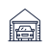•••• Slopes access :
• ski-in (-50 m)
• Rooms :
• 2 rooms
• 2 rooms + cabin(s)
• Total surface (sq.m) :
• 45 sq.m
••••• Slopes access :
• ski-in (-50 m)
• Rooms :
• 3 rooms
• Total surface (sq.m) :
• 60 sq.m
• Slopes access :
• ski-in (-50 m)
• Rooms :
• 3 rooms
• Total surface (sq.m) :
• 55 sq.m
•••••• Slopes access :
• ski-in (-50 m)
• Rooms :
• Studio
• Studio + cabin(s)
• Total surface (sq.m) :
• 22 sq.m
••••• Slopes access :
• ski-in (-50 m)
• Rooms :
• 2 rooms
• 2 rooms + cabin(s)
• Total surface (sq.m) :
• 28 sq.m
•••• Slopes access :
• ski-in (-50 m)
• Rooms :
• Studio + cabin(s)
• Total surface (sq.m) :
• 36 sq.m
••••••• Slopes access :
• ski-in (-50 m)
• Rooms :
• 4 rooms
• Total surface (sq.m) :
• 48 sq.m
•••• Slopes access :
• ski-in (-50 m)
• Rooms :
• 2 rooms + cabin(s)
• 3 rooms
• Total surface (sq.m) :
• 53-68 sq.m
•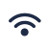•••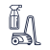••••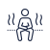•